# Mathematics Notes

Choose from our range of Mathematics Notes and revision materials, all prepared by top students during their studies.*

Name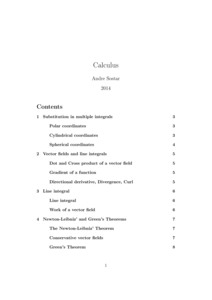Very compact and good overview of whole calculus III course covering the most important methods and formulas for success, plus a brief overview of Calculus III. Also included are some important methods and theorems, and Calculus III exercises with sol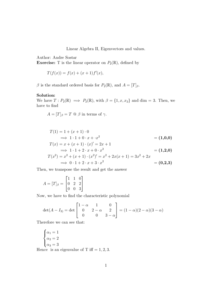Linear Algebra II most important topic eigenvectors. Exercise on finding basis, eigenvalues, eigenvectors, diagonalization. Plus exercise: Gram Schmidt process.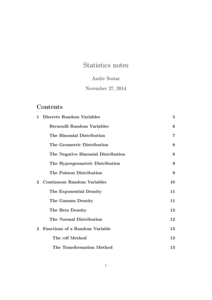Good material for last minute revision for a good grade. Also conducts all the most important facts, formulas and facts from the probability.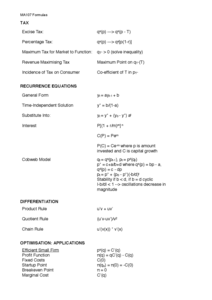Formula sheet with all necessary formulas for exam and step-by-step methods on how to answer specific types of questions (e.g. how to solve a second order differential equation).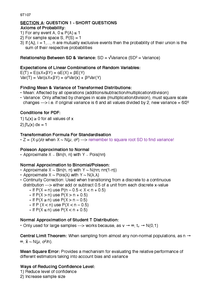Included all key formulas and definitions required for exam. Breaks down questions into types as exam follows very specific format (e.g. 4th question always examines conditional probability) with explanations of how to solve them and how marks are all

### Filter Your Mathematics Notes To A Specific University### 75K+

Customers### 98.9%

Satisfaction